# GRE Quant: The Difference of Squares

If you’ve been studying GRE for some time, you’ve very likely encountered the following: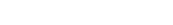.

You may, however, only seen the following equation in the context of algebra. Nevertheless, the formula above applies to number properties. Let’s take a look.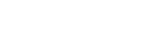While you may be tempted to make a mad dash at it, calculating each of the squares, there is an easier way. Think of the ‘16’ as the ‘x’ and the ‘15’ as the ‘y’. Using the equation above we get: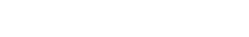.

Wow, that was much easier than figuring out the squares of both ‘16’ and ’15.’ Now, let’s try it for the second pair: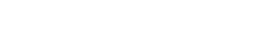That leaves us with (29)(31). I know, you may be balking at my nifty little formula, thinking you still need to do some tedious multiplication. But despair not! We can still use the difference of squares formula: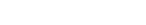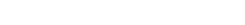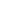is simply 3 x 3 add two zeroes: 900. Then we subtract the one and we get 899.

Next our two practice questions. The first question is not very different from the one above. The second one is more challenging and involves exponents.

## Practice Questions

1.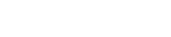?

1. 4
2. 36
3. 38
4. 76
5. 224

2.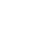=

1. 7
2. 25
3. 156
4. 175
5. 216

## Explanations:

1.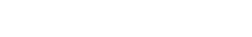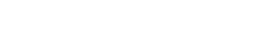76 – 72 = 4, Answer (A).

2.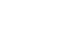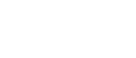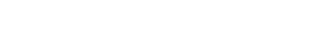.

## Author

•Chris Lele is the Principal Curriculum Manager (and vocabulary wizard) at Magoosh. Chris graduated from UCLA with a BA in Psychology and has 20 years of experience in the test prep industry. He's been quoted as a subject expert in many publications, including US News, GMAC, and Business Because. In his time at Magoosh, Chris has taught countless students how to tackle the GRE, GMAT, SAT, ACT, MCAT (CARS), and LSAT exams with confidence. Some of his students have even gone on to get near-perfect scores. You can find Chris on YouTube, LinkedIn, Twitter and Facebook!